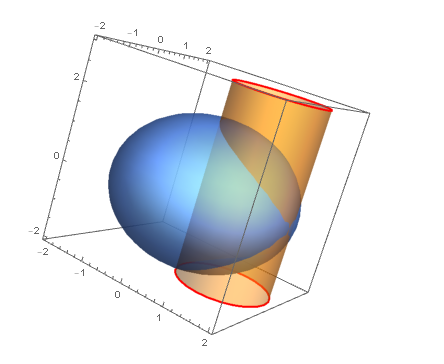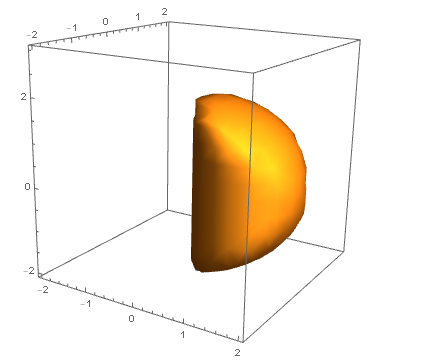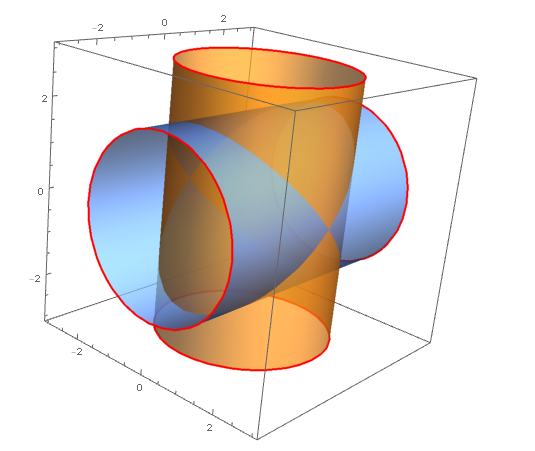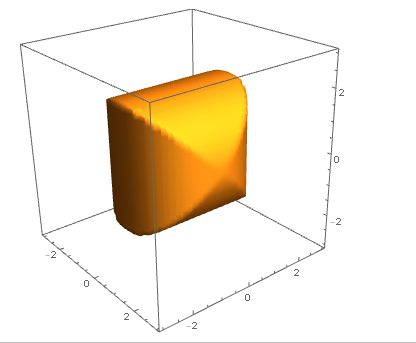# 圆柱与球相交体体积计算及扩展问题2017年12月3日20:44:38

2 1157字阅读3分51秒

## 问题解决

1. ContourPlot3D[{x^2 + y^2 == 2 x, x^2 + y^2 + z^2 == 4}, {x, -2,2}, {y, -2, 2}, {z, -2, 3},
2. ContourStyle -> {{Opacity[.5]}, {Opacity[0.7]}},
3. BoundaryStyle -> Directive[Red, Thick],
4. Mesh->None
5. ]1. r = RegionPlot3D[ x^2 + y^2 <= 2 x && x^2 + y^2 + z^2 <= 4, {x, -2, 2}, {y, -2,  2}, {z, -2, 3}, Mesh->None]1. Integrate[Boole[x^2 + y^2 <= 2 x && x^2 + y^2 + z^2 <= 4], {x, -2, 2}, {y, -2,2}, {z, -2, 3}];
2. >>16/9 (-4 + 3 Pi)

## 问题拓展--圆柱与圆柱相交

1. ContourPlot3D[{x^2 + y^2 == 4, x^2 + z^2 == 4}, {x, -3, 3}, {y, -3,3}, {z, -3, 3},
2. ContourStyle -> {{Opacity[.7]}, {Opacity[0.7]}},
3. BoundaryStyle -> Directive[Red, Thick],
4. BoxRatios -> {1, 1, 1},
5. Mesh -> None
6. ]1. RegionPlot3D[
2. x^2 + y^2 <= 4 && x^2 + z^2 <= 4, {x, -3, 3}, {y, -3, 3}, {z, -3, 3},
3. PerformanceGoal -> "Quality",
4. PlotPoints -> 50,
5. Mesh -> None]• 微信公众号
• 关注微信公众号
•• QQ群
• 我们的QQ群号
•• 本文由 发表于 2017年12月3日20:44:38
• 转载请务必保留本文链接：https://mathpretty.com/8693.html